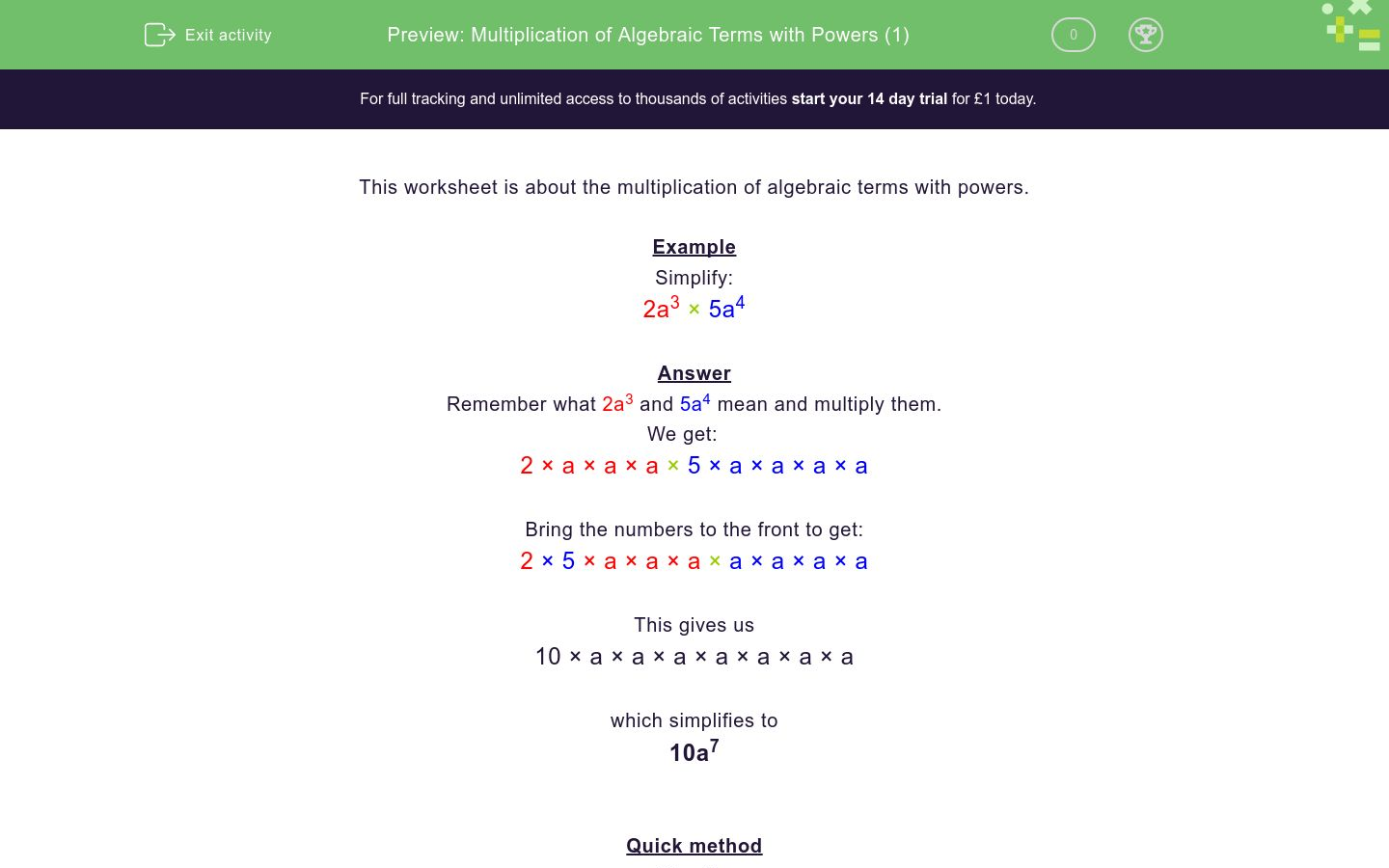# Multiplication of Algebraic Terms with Powers (1)

In this worksheet, students must multiply algebraic terms.Key stage:  KS 3

Curriculum topic:  Algebra

Curriculum subtopic:  Simplify Algebraic Expressions to Maintain Equivalence

Difficulty level:### QUESTION 1 of 10

This worksheet is about the multiplication of algebraic terms with powers.

Example

Simplify:

2a3 × 5a4

Remember what 2a3 and 5a4 mean and multiply them.

We get:

2 × a × a × a × 5 × a × a × a × a

Bring the numbers to the front to get:

2 × 5 × a × a × a × a × a × a × a

This gives us

10 × a × a × a × a × a × a × a

which simplifies to

10a7

Quick method

Simplify:

2a3 × 5a4

Multiply the numbers (2 × 5 = 10).

Add the indices of identical letters (3 + 4 = 7).

Simplify:

2a3 × 3a4

6a12

5a7

6a7

Simplify:

2a3 × 3a2

6a5

5a6

6a6

Simplify:

2b3 × 2b3

6b33

4b9

4b6

Simplify:

5b3 × 2b3

10b6

10b9

7b6

Simplify:

5c3 × 4c4

20c12

20c7

9c12

Simplify:

5c3 × 3c

15c4

8c4

15c3

Simplify:

2a6 × 4a4

6a10

8a10

8a24

Simplify:

7b7 × 3b3

21b21

10b21

21b10

Simplify:

4c4 × 4c4

16c8

8c8

16c16

Simplify:

4d4 × d6

4d24

4d10

d10

• Question 1

Simplify:

2a3 × 3a4

6a7
EDDIE SAYS
2 × 3 × a × a × a × a × a × a × a
• Question 2

Simplify:

2a3 × 3a2

6a5
EDDIE SAYS
2 × 3 × a × a × a × a × a
• Question 3

Simplify:

2b3 × 2b3

4b6
EDDIE SAYS
2 × 2 × b × b × b × b × b × b
• Question 4

Simplify:

5b3 × 2b3

10b6
EDDIE SAYS
5 × 2 × b × b × b × b × b × b
• Question 5

Simplify:

5c3 × 4c4

20c7
EDDIE SAYS
5 × 4 × c × c × c × c × c × c × c
• Question 6

Simplify:

5c3 × 3c

15c4
EDDIE SAYS
5 × 3 × c × c × c × c
• Question 7

Simplify:

2a6 × 4a4

8a10
EDDIE SAYS
2 × 4 × a × a × a × a × a × a × a × a × a × a
• Question 8

Simplify:

7b7 × 3b3

21b10
EDDIE SAYS
7 × 3 × b × b × b × b × b × b × b × b × b × b
• Question 9

Simplify:

4c4 × 4c4

16c8
EDDIE SAYS
4 × 4 × c × c × c × c × c × c × c × c
• Question 10

Simplify:

4d4 × d6